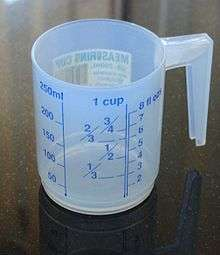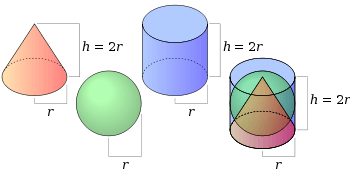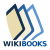# Volume

For other uses, see Volume (disambiguation).A measuring cup can be used to measure volumes of liquids. This cup measures volume in units of cups, fluid ounces, and millilitres.

Volume is the quantity of three-dimensional space enclosed by a closed surface, for example, the space that a substance (solid, liquid, gas, or plasma) or shape occupies or contains. Volume is often quantified numerically using the SI derived unit, the cubic metre. The volume of a container is generally understood to be the capacity of the container, i. e. the amount of fluid (gas or liquid) that the container could hold, rather than the amount of space the container itself displaces.

Three dimensional mathematical shapes are also assigned volumes. Volumes of some simple shapes, such as regular, straight-edged, and circular shapes can be easily calculated using arithmetic formulas. Volumes of a complicated shape can be calculated by integral calculus if a formula exists for the shape's boundary. Where a variance in shape and volume occurs, such as those that exist between different human beings, these can be calculated using three-dimensional techniques such as the Body Volume Index. One-dimensional figures (such as lines) and two-dimensional shapes (such as squares) are assigned zero volume in the three-dimensional space.

The volume of a solid (whether regularly or irregularly shaped) can be determined by fluid displacement. Displacement of liquid can also be used to determine the volume of a gas. The combined volume of two substances is usually greater than the volume of one of the substances. However, sometimes one substance dissolves in the other and the combined volume is not additive.

In differential geometry, volume is expressed by means of the volume form, and is an important global Riemannian invariant. In thermodynamics, volume is a fundamental parameter, and is a conjugate variable to pressure.

## UnitsVolume measurements from the 1914 The New Student's Reference Work.
Approximate conversion to millilitres:
Imperial U.S. liquid U.S. dry
Gill 142 mL 118 mL 138 mL
Pint 568 mL 473 mL 551 mL
Quart 1137 mL 946 mL 1101 mL
Gallon 4546 mL 3785 mL 4405 mL

Any unit of length gives a corresponding unit of volume: the volume of a cube whose sides have the given length. For example, a cubic centimetre (cm3) is the volume of a cube whose sides are one centimetre (1 cm) in length.

In the International System of Units (SI), the standard unit of volume is the cubic metre (m3). The metric system also includes the litre (L) as a unit of volume, where one litre is the volume of a 10-centimetre cube. Thus

1 litre = (10 cm)3 = 1000 cubic centimetres = 0.001 cubic metres,

so

1 cubic metre = 1000 litres.

Small amounts of liquid are often measured in millilitres, where

1 millilitre = 0.001 litres = 1 cubic centimetre.

Various other traditional units of volume are also in use, including the cubic inch, the cubic foot, the cubic mile, the teaspoon, the tablespoon, the fluid ounce, the fluid dram, the gill, the pint, the quart, the gallon, the minim, the barrel, the cord, the peck, the bushel, and the hogshead.

## Related terms

Capacity is defined by the Oxford English Dictionary as "the measure applied to the content of a vessel, and to liquids, grain, or the like, which take the shape of that which holds them". (The word capacity has other unrelated meanings, as in e.g. capacity management.) Capacity is not identical in meaning to volume, though closely related; the capacity of a container is always the volume in its interior. Units of capacity are the SI litre and its derived units, and Imperial units such as gill, pint, gallon, and others. Units of volume are the cubes of units of length. In SI the units of volume and capacity are closely related: one litre is exactly 1 cubic decimetre, the capacity of a cube with a 10 cm side. In other systems the conversion is not trivial; the capacity of a vehicle's fuel tank is rarely stated in cubic feet, for example, but in gallons (a gallon fills a volume of 0.1605 cu ft).

The density of an object is defined as the ratio of the mass to the volume. The inverse of density is specific volume which is defined as volume divided by mass. Specific volume is a concept important in thermodynamics where the volume of a working fluid is often an important parameter of a system being studied.

The volumetric flow rate in fluid dynamics is the volume of fluid which passes through a given surface per unit time (for example cubic meters per second [m3 s−1]).

## Volume in calculus

Further information: Volume element

In calculus, a branch of mathematics, the volume of a region D in R3 is given by a triple integral of the constant functionand is usually written as:The volume integral in cylindrical coordinates isand the volume integral in spherical coordinates (using the convention for angles withas the azimuth andmeasured from the polar axis (see more on conventions)) has the form## Volume formulas

Shape Volume formula Variables
Cubea = length of any side (or edge)
Cylinderr = radius of circular face, h = height
PrismB = area of the base, h = height
Rectangular prisml = length, w = width, h = height
Triangular prismb = base length of triangle, h = height of triangle, l = length of prism or distance between the triangular bases
Spherer = radius of sphere
which is the integral of the surface area of a sphere
Ellipsoida, b, c = semi-axes of ellipsoid
Torusr = minor radius (radius of the tube), R = major radius (distance from center of tube to center of torus)
PyramidB = area of the base, h = height of pyramid
Square pyramids = side length of base, h = height
Rectangular pyramidl = length, w = width, h = height
Coner = radius of circle at base, h = distance from base to tip or height
Tetrahedronedge lengthParallelepipeda, b, and c are the parallelepiped edge lengths, and α, β, and γ are the internal angles between the edges
Any volumetric sweep
(calculus required)h = any dimension of the figure,
A(h) = area of the cross-sections perpendicular to h described as a function of the position along h. a and b are the limits of integration for the volumetric sweep.
(This will work for any figure if its cross-sectional area can be determined from h).
Any rotated figure (washer method)
(calculus required)andare functions expressing the outer and inner radii of the function, respectively.

### Volume ratios for a cone, sphere and cylinder of the same radius and heightA cone, sphere and cylinder of radius r and height h

The above formulas can be used to show that the volumes of a cone, sphere and cylinder of the same radius and height are in the ratio 1 : 2 : 3, as follows.

Let the radius be r and the height be h (which is 2r for the sphere), then the volume of cone isthe volume of the sphere iswhile the volume of the cylinder isThe discovery of the 2 : 3 ratio of the volumes of the sphere and cylinder is credited to Archimedes.

### Volume formula derivations

#### Sphere

The volume of a sphere is the integral of an infinite number of infinitesimally small circular disks of thickness dx. The calculation for the volume of a sphere with center 0 and radius r is as follows.

The surface area of the circular disk is.

The radius of the circular disks, defined such that the x-axis cuts perpendicularly through them, isorwhere y or z can be taken to represent the radius of a disk at a particular x value.

Using y as the disk radius, the volume of the sphere can be calculated asNowCombining yieldsThis formula can be derived more quickly using the formula for the sphere's surface area, which is. The volume of the sphere consists of layers of infinitesimally thin spherical shells, and the sphere volume is equal to=#### Cone

The cone is a type of pyramidal shape. The fundamental equation for pyramids, one-third times base times altitude, applies to cones as well.

However, using calculus, the volume of a cone is the integral of an infinite number of infinitesimally thin circular disks of thickness dx. The calculation for the volume of a cone of height h, whose base is centered at (0,0,0) with radius r, is as follows.

The radius of each circular disk is r if x = 0 and 0 if x = h, and varying linearly in between—that is,The surface area of the circular disk is thenThe volume of the cone can then be calculated asand after extraction of the constants:Integrating gives us## Volume in differential geometry

Main article: Volume form

In differential geometry, a branch of mathematics, a volume form on a differentiable manifold is a differential form of top degree (i.e. whose degree is equal to the dimension of the manifold) that is nowhere equal to zero. A manifold has a volume form if and only if it is orientable. An orientable manifold has infinitely many volume forms, since multiplying a volume form by a non-vanishing function yields another volume form. On non-orientable manifolds, one may instead define the weaker notion of a density. Integrating the volume form gives the volume of the manifold according to that form.

Any oriented Riemannian (or pseudo-Riemannian) manifold has a natural volume (or pseudo volume) form. In local coordinates, it can be expressed aswhere theare the 1-forms providing an oriented basis for the cotangent bundle of the n-dimensional manifold. Here,is the absolute value of the determinant of the matrix representation of the metric tensor on the manifold.

## Volume in thermodynamics

In thermodynamics, the volume of a system is an important extensive parameter for describing its thermodynamic state. The specific volume, an intensive property, is the system's volume per unit of mass. Volume is a function of state and is interdependent with other thermodynamic properties such as pressure and temperature. For example, volume is related to the pressure and temperature of an ideal gas by the ideal gas law.

## References

1. "Your Dictionary entry for "volume"". Retrieved 2010-05-01.
2. One litre of sugar (about 970 grams) can dissolve in 0.6 litres of hot water, producing a total volume of less than one litre. "Solubility". Retrieved 2010-05-01. Up to 1800 grams of sucrose can dissolve in a liter of water.
3. "General Tables of Units of Measurement". NIST Weights and Measures Division. Retrieved 2011-01-12.
4. "capacity". Oxford English Dictionary (3rd ed.). Oxford University Press. September 2005. (Subscription or UK public library membership required.)
5. "density". Oxford English Dictionary (3rd ed.). Oxford University Press. September 2005. (Subscription or UK public library membership required.)
6. Coxeter, H. S. M.: Regular Polytopes (Methuen and Co., 1948). Table I(i).
7. Rorres, Chris. "Tomb of Archimedes: Sources". Courant Institute of Mathematical Sciences. Retrieved 2007-01-02.The Wikibook Geometry has a page on the topic of: Perimeters, Areas, VolumesThe Wikibook Calculus has a page on the topic of: Volume